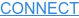Mortal humans often think of Simple Random Sampling (SRS) as a sampling design where all elements of the sample have equal probability of being selected. Unfortunately, although this is a necessary condition for SRS, it is not a sufficient condition.

For example, let’s say that there are 100 pieces of chocolates in our kitchen cabinet. We go to the cabinet and take out the first chocolate, roll a die, and eat the chocolate if it is a 6, and put it aside if it is not. Then we take the second one and repeat the procedure, and then the third one, and so on. Do all chocolates have equal probability of being eaten? Yes, one sixth. How many chocolates are we going to eat? We do not know this in advance. It could be zero, it could be 100, or anything in between: the sample size is not fixed. This is not SRS, this is called a Bernoulli sampling design.

Consider another example where we have a population of 100 Kindergarten students: 50 girls and 50 boys. We first toss a coin (50%-50% chance) to decide whether we are going to select boys or girls into our sample, and then randomly select 10 students from either the boys’ or the girls’ group. Is this a fixed sample size design? Yes, all our potential samples will have 10 students. Do all students have equal probability of being selected. Yes, 10%, because we are selecting 10 students from 100. Is this SRS? No, this is a special case of stratified sampling. We don’t call this SRS, because the chances of obtaining any given combination of 10 students are not equal. In particular, the chances of some combinations, those that include both boys and girls, are zero.

## So What is a Simple Random Sample?

A Simple Random Sample is a sample where:

1. Sample size is a fixed number of elements, n.

1. Each possible combination of the n elements in the population has the same chance of being selected as the sample.

1. No single element can be sampled twice. Samples where an element can be sampled twice are called samples with replacement.

There are valid statistical samples where any of these three conditions fail to hold, and we will discuss them in future posts, but these samples are not called Simple Random Samples.

## How Should You Select a Sample Using SRS?

In order to select a sample from a population using SRS, we have to make sure that the sample size will be exactly what we want it to be (unlike in the first example with the chocolates), and that the selection procedure (the randomization) does not depend on the characteristics of the population (unlike in the second example with the Kindergarten students).

We typically want a sample that can be “replicated,” such that someone can verify that we pulled it correctly. The first step in such a case would be to sort the data by a unique identifier, e.g., a student’s social security number or student id. The second step would be to assign a random number seed to the software’s random number generator. At Summit, we typically use the date in the following format: YYYYMMDD, which allows for little manipulation of the seed. The third step is to assign random numbers. The fourth step is to sort by random numbers, and the final step is to select the first n elements into the sample, where n is the sample size.

An SRS selected this way can be completely replicated by anyone with the data and knowledge of the random number seed and software used. This allows for verification that no mistakes were made in the sampling procedure and that no ‘cherry-picking’ of sampled items was performed.

This post was written with the help of Sejla Karalic and Dr. Alan Salzberg.